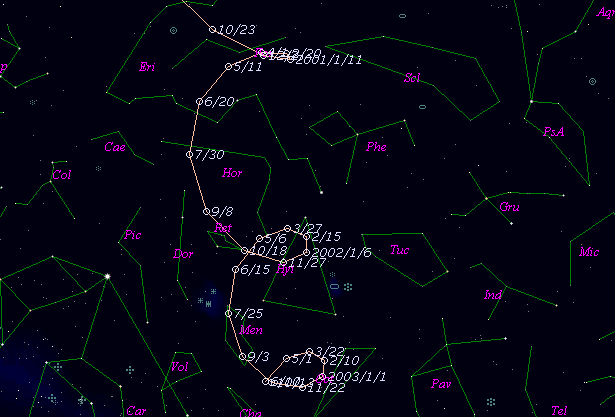# \$B%j%K%"WB@1(B

C/1999 T3 ( LINEAR )###\$B%W%m%U%#!<%k(B

 \$BH/8+F|(B 1999\$BG/(B10\$B7n(B3\$BF|(B \$BH/8+8wEY(B 18.3\$BEy(B \$BH/8+ Lincoln Laboratory Near-Earth Asteroid Research project

###\$B###\$B50F;MWAG(B

```   The following improved orbital elements by Kenji Muraoka, are
from 174 observations 1999 Oct. 3 to 2000 Feb. 4, perturbations
by 9 Planets, Moon and 5 minor planets were taken into account.
The mean residual is +/- 0.64 arc seconds.

Epoch  =  2000 Sep. 13.0  TT       JDT = 2451800.5
T  =  2000 Sep.  1.68174       +/- 0.06388 (m.e.) TT
Peri. =  211.33740                +/- 0.00555
Node  =  223.51187                +/- 0.00194   (2000.0)
Incl. =  104.75245                +/- 0.00524
q  =    5.3651610              +/- 0.0002801 AU
e  =    0.9969272              +/- 0.0001188
1/a  =   +0.0005727              +/- 0.0000221 1/AU
orig. 1/a  =   +0.000234
fut.  1/a  =   +0.001148
```

###\$B@1?^(B###\$B8wEYJQ2=(B

```        m1 = 6.5 + 5 log\$B&\$(B + 10.0 log r
```##### \$B50F;MWAG\$OB<2,7r<#;a\$N7W;;\$K\$h\$k\$b\$N\$G\$9!#(B \$B@1?^\$O(B StellaNavigator Ver.2.0 for Windows (\$B%"%9%H%m%"!<%D(B \$BJTCx(B / \$B%"%9%-!<=PHG6I4)(B) \$B\$G:n@.\$7\$?\$b\$N\$G\$9!#(B \$B8wEY%0%i%U\$O(BComet for Windows\$B\$G:n@.\$7\$?\$b\$N\$G\$9!#(B